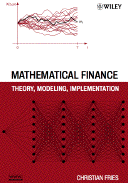Mathematical Finance: Theory, Modeling, Implementation

# Suggestions for Lecturers

In the following you find some suggestions for lectures based on the book. The book is sufficient for two semesters, each having 14 units of 90 min lecture.

## Lecture: Mathematical Finance Introduction

The lecture tries to be fully self-contained, repeating some basic material from probability theory. However, the lecture does not give many proof. Proofs are only given if they show important aspects or techniques (like the derivation of the Black-Scholes formula). Each session takes approximately 90 min.

### Session 0: Introduction (optional)

Introduce the idea of replication following Section 3.1.
• Introduce the static hedge.
• Motivating the need for a stochastic model for a dynamic hedge.
• Motivate the idea of a change of measure using Section 3.1.2.

### Session 1: Foundations from Probability Theory

Section 2.1 to 2.3

### Session 2: Building Stochastic Processes

Section 2.4 to 2.6

### Session 3: Ito Calculus, Martingales, Martingale Representation

Section 2.6.1 to 2.9

Section 3.2

Chapter 4

Chapter 8

Chapter 9

### Session 8: Simple Interest Rate Options

Chapter 10: The Black Model for a Caplet

### Session 9: LIBOR Market Model: Derivation of the Drift, Model Parameters

Chapter 19, Section 19.1 to 19.2

### Session 10: LIBOR Market Model: Model Properties and Model Intuition

Chapter 19, Section 19.4; Chapter 25

### Session 11: Discretization and Monte-Carlo Simulation

Chapter 13, Application to Black-Scholes Model and/or LIBOR Market Model

### Session 12: Pricing Bermudan Option in a Monte Carlo Simulation

Section 15.1 to 15.4: Bermudan Options and the Backward Algorithm

### Session 13: Pricing Bermudan Option in a Monte Carlo Simulation: Estimating the Excercise Criteria

Section 15.5 to 15.8, 15.10

### Session 14: Sensitivities of Monte Carlo Prices: Problem Description

Section 17.1 to 17.3

### Session 15: Sensitivities of Monte Carlo Prices: Problem Solutions

Section 17.4 to 17.8